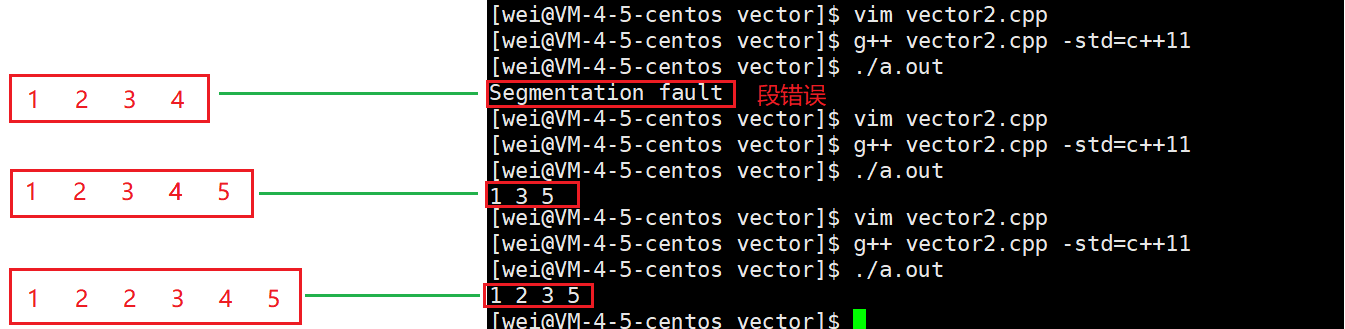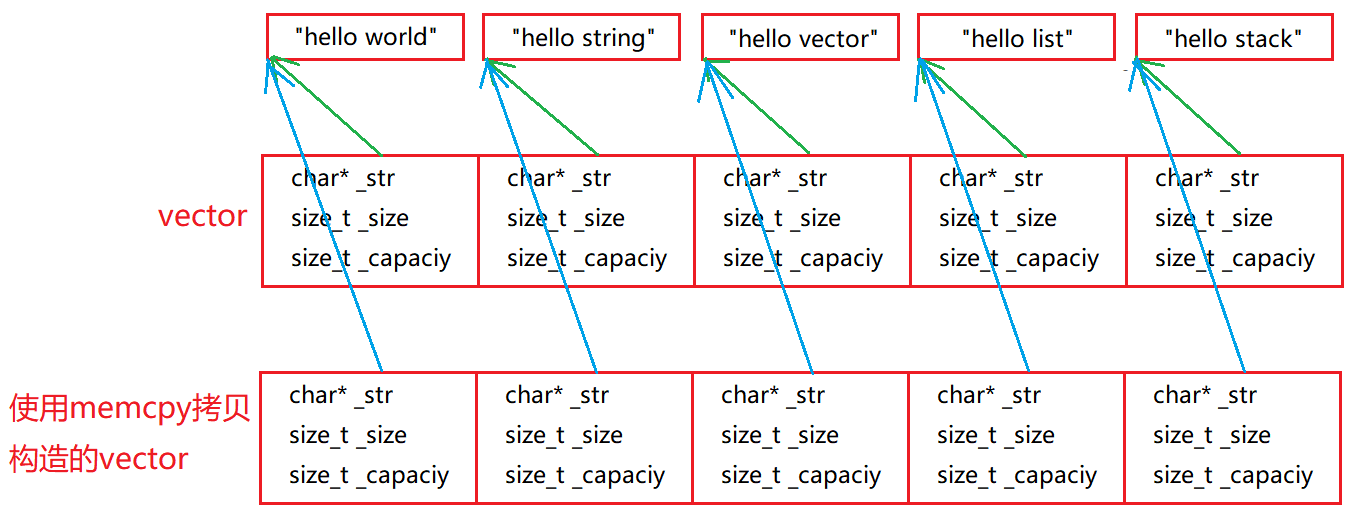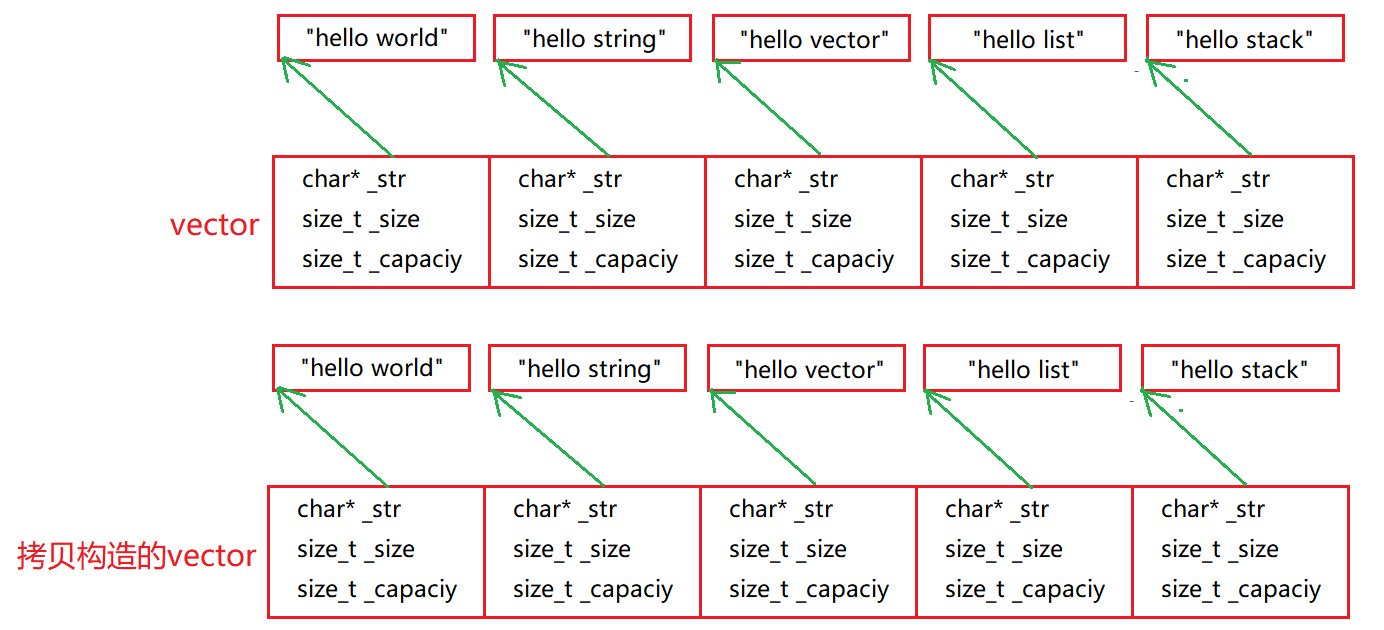# C++中vector迭代器失效與深淺拷貝問題詳析

## 一、vector迭代器失效問題

### 1. insert迭代器失效

```void insert(iterator pos, const T& x)
{
//檢測參數合法性
assert(pos >= _start);
assert(pos <= _finish);
//檢測是否需要擴容
if (_finish == _endofstorage)
{
size_t newcapcacity = capacity() == 0 ? 4 : capacity() * 2;
reserve(newcapcacity);
}
//挪動數據
iterator end = _finish - 1;
while (end >= pos)
{
*(end + 1) = *end;
end--;
}
//插入指定的數據
*pos = x;
_finish++;
}
```

insert的迭代器失效分為兩大類：

#### 1.1.擴容導致野指針```void insert(iterator pos, const T& x)
{
//檢測參數合法性
assert(pos >= _start);
assert(pos <= _finish);
//檢測是否需要擴容,擴容以後pos就失效瞭，需要更新一下
if (_finish == _endofstorage)
{
size_t n = pos - _start;//計算pos和start的相對距離
size_t newcapcacity = capacity() == 0 ? 4 : capacity() * 2;
reserve(newcapcacity);
// 擴容會導致pos迭代器失效，需要更新處理一下
pos = _start + n;//防止迭代器失效，要讓pos始終指向與_start間距n的位置
}
//挪動數據
iterator end = _finish - 1;
while (end >= pos)
{
*(end + 1) = *end;
end--;
}
//插入指定的數據
*pos = x;
_finish++;
}
```

#### 1.2.迭代器指向位置意義改變1. 和上面的錯誤一樣，首先it是指向原來的空間，當insert插入新元素時會發生擴容，原來的舊數據被拷貝到瞭新空間上，並且釋放舊空間，這也就意味著舊空間已經被操作系統回收，而it一直是指向舊空間的，隨後遍歷it時就非法訪問野指針，也就失效瞭。形參的改變不會影響實參，即使你內部pos的指向改變瞭，但是並不會影響我外部的it。所以我們仍然無法通過it去訪問元素。
2. 為瞭解決上面的錯誤，有人可能會說提前reserve開辟足夠大的空間即可避免發生野指針的現象，但是又出現瞭一個新的問題，看圖：```iterator insert(iterator pos, const T& x)
{
//檢測參數合法性
assert(pos >= _start);
assert(pos <= _finish);
//檢測是否需要擴容,擴容以後pos就失效瞭，需要更新一下
if (_finish == _endofstorage)
{
size_t n = pos - _start;//計算pos和start的相對距離
size_t newcapcacity = capacity() == 0 ? 4 : capacity() * 2;
reserve(newcapcacity);
// 擴容會導致pos迭代器失效，需要更新處理一下
pos = _start + n;//防止迭代器失效，要讓pos始終指向與_start間距n的位置
}
//挪動數據
iterator end = _finish - 1;
while (end >= pos)
{
*(end + 1) = *end;
end--;
}
//插入指定的數據
*pos = x;
_finish++;

return pos;
}
```

```//在所有的偶數前面插入2
void test_vector3()
{
vector<int> v;
v.reserve(10);
v.push_back(1);
v.push_back(2);
v.push_back(3);
v.push_back(4);

vector<int>::iterator it = find(v.begin(), v.end(), 1);
while (it != v.end())
{
if (*it % 2 == 0)
{
it = v.insert(it, 20);
}
it++;
}
for (auto e : v)
{
cout << e << " ";
}
cout << endl;
}
```

#### 1.3.windows下VS中標準庫和Linux下g++中標準庫對insert迭代器失效的處理

VS：Linux：### 2. erase迭代器失效

```// 返回刪除數據的下一個數據
// 方便解決:一邊遍歷一邊刪除的迭代器失效問題
void erase(iterator pos)
{
assert(pos >= _start);
assert(pos < _finish);
//從pos + 1的位置開始往前覆蓋，即可完成刪除pos位置的值
iterator begin = pos + 1;
while (begin < _finish)
{
*(begin - 1) = *begin;
}
_finish--;
}
```
• erase的失效都是意義變瞭，或者不在有效訪問數據的有效范圍內
• 一般不會使用縮容的方案，那麼erase的失效，一般也不存在野指針的失效

#### 2.1.迭代器失效指向位置意義改變

```void test_vector2()
{
cpp::vector<int> v;
//v.reserve(10);
v.push_back(1);
v.push_back(2);
v.push_back(3);
v.push_back(4);
cout << v.size() << ":" << v.capacity() << endl;
vector<int>::iterator it = find(v.begin(), v.end(), 2);
if (it != v.end())
{
v.erase(it);
}
cout << *it << endl; // 讀
(*pos)++; // 寫
cout << *it << endl << endl;
cout << v.size() << ":" << v.capacity() << endl;
for (auto e : v)
{
cout << e << " ";
}
}
```#### 2.2.windows下VS中標準庫和Linux下g++中標準庫對erase迭代器失效的處理

VS下：VS環境下檢查非常嚴格， 直接強制檢查斷言錯誤。

Linux下：1. erase(pos)以後pos失效瞭，pos的意義變瞭，但是在不同平臺下面對於訪問pos的反應是不一樣的，我們用的時候要以失效的角度去看待此問題。
2. 對於insert和erase造成迭代器失效問題，linux的g++平臺檢查並不是很嚴格，基本靠操作系統本身野指針越界檢查機制。windows下VS系列檢查更嚴格一些，使用一些強制檢查機制，意義變瞭可能會檢查出來。
3. 雖然g++對於迭代器失效檢查時是並不嚴格，但是套在實際場景中，迭代器意義變瞭，也會出現各種問題。

• 1 2 3 4
• 1 2 3 4 5
• 1 2 2 3 4 5
```void test_vector4()
{
//刪除所有的偶數
std::vector<int> v;
//v.reserve(10);
// 第一組測試用例:
v.push_back(1);
v.push_back(2);
v.push_back(3);
v.push_back(4);
auto it = v.begin();
while (it != v.end())
{
if (*it % 2 == 0)
{
v.erase(it);
}
it++;
}
for (auto e : v)
{
cout << e << " ";
}
}
``````// 返回刪除數據的下一個數據
// 方便解決:一邊遍歷一邊刪除的迭代器失效問題
void erase(iterator pos)
{
assert(pos >= _start);
assert(pos < _finish);
//從pos + 1的位置開始往前覆蓋，即可完成刪除pos位置的值
iterator begin = pos + 1;
while (begin < _finish)
{
*(begin - 1) = *begin;
}
_finish--;
return pos;
}
```

```void test4()
{
//刪除所有的偶數
std::vector<int> v;
//v.reserve(10);
v.push_back(1);
v.push_back(2);
v.push_back(3);
v.push_back(4);
auto it = v.begin();
while (it != v.end())
{
if (*it % 2 == 0)
{
it = v.erase(it);
}
else
{
it++;
}
}
for (auto e : v)
{
cout << e << " ";
}
}
```

erase刪除pos位置元素後，pos位置之後的元素會往前移動，沒有導致底層空間的改變，理論上講迭代器不會失效，但是如果pos位置剛好是最後一個元素，刪完之後pos剛好是end的位置，而end的位置是沒有有效元素的，那麼pos就失效瞭。因此刪除vector中任意位置元素時，vs均認為該位置上迭代器失效瞭！也就是說vector刪除一定會導致迭代器失效。

### 3.迭代器失效總結

vector迭代器失效有2種

1、擴容，導致野指針失效

2、迭代器指向的位置意義變瞭

1. 對於insert和erase造成迭代器失效問題，linux g++平臺檢查很松懈，基本依靠操作系統自身野指針越界檢查機制，windows下vs系列檢查更嚴格，使用一些強制檢查機制，意義變瞭也可能會檢查出來。
2. 雖然g+＋對於erase迭代器失效檢查時非常雞肋的，但是套在實際場景中，迭代器意義變瞭，也會出現各種問題，所以我們要有正確處理迭代器失效的方式,比如用函數返回值來更新迭代器。
3. windows下vs系列對意義失效的檢查很雙標，由insert函數引起的意義失效檢查不出來，而且可以訪問pos位置，但是由erase函數引起的意義失效卻檢查很嚴格，絲毫不準訪問pos位置。但是Linux平臺下都檢查不出來，都可以訪問pos位置。

## 二、深淺拷貝問題

### 1.拷貝構造淺拷貝問題

```// 拷貝構造 v1(v)
// 傳統寫法
vector(const vector<T>& v)
:_start(nullptr)
,_finish(nullptr)
,_endofstorage(nullptr)
{
_start = new T[v.capacity()]; // 開辟一塊和v大小相同的空間
memcpy(_start, v._start, sizeof(T) * v.size()); //error
_finish = _start + v.size();
_endofstorage = _start + v.capacity();
}
``````for (size_t i = 0; i < v.size(); i++)
{
_start[i] = v[i];
}
``````// 拷貝構造 v1(v)
// 傳統寫法
vector(const vector<T>& v)
:_start(nullptr)
,_finish(nullptr)
,_endofstorage(nullptr)
{
_start = new T[v.capacity()]; // 開辟一塊和v大小相同的空間
for (size_t i = 0; i < v.size(); i++)
{
_start[i] = v[i];
}
//memcpy(_start, v._start, sizeof(T) * v.size()); //error
_finish = _start + v.size();
_endofstorage = _start + v.capacity();
}
```

### 2.擴容淺拷貝問題

```namespace vector_realize
{
/* class Solution {
public:
// 核心思想：找出楊輝三角的規律，發現每一行頭尾都是1，中間第[j]個數等於上一行[j-1]+[j]
vector<vector<int>> generate(int numRows) {
vector<vector<int>> vv;
vv.resize(numRows);// 先開辟楊輝三角的空間
for (size_t i = 0; i < vv.size(); ++i)
{
vv[i].resize(i + 1, 0);
vv[i] = vv[i][vv[i].size() - 1] = 1;// 每一行的第一個和最後一個都是1
}

for (size_t i = 0; i < vv.size(); ++i)
{
for (size_t j = 0; j < vv[i].size(); ++j)
{
if (vv[i][j] == 0)
{
vv[i][j] = vv[i - 1][j] + vv[i - 1][j - 1];
}
}
}

return vv;
}
};

void test_vector9()
{
vector<vector<int>> vvRet = Solution().generate(5);

for (size_t i = 0; i < vvRet.size(); ++i)
{
for (size_t j = 0; j < vvRet[i].size(); ++j)
{
cout << vvRet[i][j] << " ";
}
cout << endl;
}
cout << endl;
}*/

vector<vector<int>> vv;
vector<int> v(5, 1);
vv.push_back(v);
vv.push_back(v);
vv.push_back(v);
vv.push_back(v);
vv.push_back(v);

for (size_t i = 0; i < vv.size(); i++)
{
for (size_t j = 0; j < vv[i].size(); j++)
{
cout << vv[i][j] << " ";
}
cout << endl;
}
cout << endl;
}
``````//reserve擴容
void reserve(size_t n)
{
int oldSize = size();
if (capacity() < n)
{
// 1.開辟新空間
T* tmp = new T[n];
if (_start)
{
//2.拷貝元素
memcpy(tmp, _start, sizeof(T) * size());
//3. 釋放舊空間
delete[] _start;
}
_start = tmp;
}
// 這裡_start的地址變瞭，而_finish還是原來的位置
//_finish = _start + size(); error
_finish = _start + oldSize;
_endofstorage = _start + n;
}
```1. vector中，當T設計深淺拷貝的類型時，如：string/vector等等，我們擴容使用memcpy拷貝數據是存在淺拷貝問題。
2. memcpy是內存的二進制格式拷貝，將一段內存空間中內容原封不動的拷貝到另外一段內存空間中。
3. 如果拷貝的是自定義類型的元素，memcpy即高效又不會出錯，但如果拷貝的是自定義類型元素，並且自定義類型元素中涉及到資源管理時，就會出錯，因為memcpy的拷貝實際是淺拷貝。

reserve擴容時不使用memcpy，改成for循環來解決：

```//reserve擴容
void reserve(size_t n)
{
int oldSize = size();
if (capacity() < n)
{
// 1.開辟新空間
T* tmp = new T[n];
if (_start)
{
//2.拷貝元素
// 這裡直接用memcpy會有問題，發生淺拷貝
//memcpy(tmp, _start, sizeof(T) * size());
for (size_t i = 0; i < oldSize; i++)
{
tmp[i] = _start[i]; // 本質調用賦值運算符重載進行深拷貝
}
//3. 釋放舊空間
delete[] _start;
}
_start = tmp;
}
// 這裡_start的地址變瞭，而_finish還是原來的位置
//_finish = _start + size(); error
_finish = _start + oldSize;
_endofstorage = _start + n;
}
```